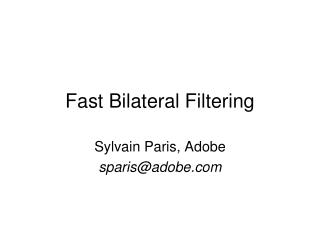DownloadDownload PresentationFast Bilateral Filtering

# Fast Bilateral Filtering

Télécharger la présentation## Fast Bilateral Filtering

- - - - - - - - - - - - - - - - - - - - - - - - - - - E N D - - - - - - - - - - - - - - - - - - - - - - - - - - -
##### Presentation Transcript

2. Manipulating the texture in a photo isa central operation in many tasks. • Let’s look at a few examples…

3. Tone Mapping[Durand 02] HDR input

4. Tone Mapping[Durand 02] output

5. Naïve Approach: Gaussian Blur BLUR HALOS smoothed(structure, large scale) residual(texture, small scale) input Gaussian Convolution

6. Impact of Blur and Halos • If the decomposition introduces blur and halos, the final result is corrupted. Sample manipulation:increasing texture(residual 3)

7. Bilateral Filter: no Blur, no Halos smoothed(structure, large scale) residual(texture, small scale) input edge-preserving: Bilateral Filter

8. input

9. increasing texturewith Gaussian convolutionH A L O S

10. increasing texturewith bilateral filterN O H A L O S

11. Traditional Denoising versus Computational Photography Edge-preserving filtering introduced for denoising. • Denoising: decompose into signal + noise • Throw away noise • Small kernels • Computational photography: decompose into base + detail • Detail is valuable • Large kernels • Bilateral filter [Aurich 95, Smith 97, Tomasi 98]

12. Objective of bilateral filtering Smooth texture Preserve edges

13. pixelintensity pixel position Illustration a 1D Image • 1D image = line of pixels • Better visualized as a plot

14. space space range normalization Definition Gaussian blur p q • only spatial distance, intensity ignored space Bilateral filter[Aurich 95, Smith 97, Tomasi 98] p range q • spatial and range distances • weights sum to 1 space

15. output    Example on a Real Image • Kernels can have complex, spatially varying shapes. input

16. Bilateral Filter is Expensive • Brute-force computation is slow (several minutes) • Two nested for loops: for each pixel, look at all pixels • Non-linear, depends on image content  no FFT, no pre-computation… • Fast approximations exist [Durand 02, Weiss 06] • Significant loss of accuracy • No formal understanding of accuracy versus speed

17. Today • We will reformulate the bilateral filter • Link with linear filtering • Fast and accurate algorithm

18. Questions ?

19. Outline • Reformulation of the BF • Fast algorithm to compute the BF • Practical implementation • Application and extension • Photographic style transfer • Bilateral grid

20. Our Strategy p weightsappliedto pixels Reformulate the bilateral filter • More complex space: • Homogeneous intensity • Higher-dimensional space • Simpler expression: mainly a convolution • Leads to a fast algorithm

21. Link with Linear Filtering1. Handling the Division p sum ofweights Handling the division with a projective space.

22. Normalizing factor as homogeneous coordinate • Multiply both sides by Formalization: Handling the Division

23. Formalization: Handling the Division • Similar to homogeneous coordinates in projective space • Division delayed until the end with Wq=1

24. Questions ?

25. 2D Gaussian Link with Linear Filtering2. Introducing a Convolution p q space range

26. Link with Linear Filtering2. Introducing a Convolution p q space x range Corresponds to a 3D Gaussian on a 2D image. Result appeared previously in [Barash 02].

27. Link with Linear Filtering2. Introducing a Convolution sum all values space-range Gaussian sum all values multiplied by kernel  convolution

28. Link with Linear Filtering2. Introducing a Convolution result of the convolution space-range Gaussian

29. Link with Linear Filtering2. Introducing a Convolution result of the convolution space-range Gaussian

30. higher dimensional functions w i w Gaussian convolution division slicing

31. Reformulation: Summary • Convolution in higher dimension • expensive but well understood (linear, FFT, etc) • Division and slicing • nonlinear but simple and pixel-wise Exact reformulation

32. Questions ?

33. Outline • Reformulation of the BF • Fast algorithm to compute the BF • Practical implementation • Application and extension • Photographic style transfer • Bilateral grid

34. Higherdimensional Homogeneousintensity • Recap: • simple operations • complex space higher dimensional functions w i w Gaussian convolution division slicing

35. Strategy:downsampledconvolution higher dimensional functions w i w D O W N S A M P L E Heavilydownsampled Gaussian convolution U P S A M P L E division slicing

36. Sampling Theorem • Sampling a signal at a least twice its smallest wavelength is enough. Not enough

37. Sampling Theorem • Sampling a signal at a least twice its smallest wavelength is enough. Not enough

38. Sampling Theorem • Sampling a signal at a least twice its smallest wavelength is enough. Enough

39. Almost onlylow freq. High freq. negligible Signal processinganalysis higher dimensional functions w i w Low-pass filter Gaussian convolution division slicing

40. “Safe” downsampling higher dimensional functions w i w D O W N S A M P L E Almost noinformationloss Gaussian convolution U P S A M P L E division slicing

41. Fast Convolution by Downsampling • Downsampling cuts frequencies above Nyquist limit (half the sampling rate) • Less data to process • But introduces error • Evaluation of the approximation • Efficient implementation

42. finer sampling Accuracy versus Running Time 1 secondinstead ofseveral minutes • Finer sampling increases accuracy. • More precise than previous work. Accuracy as function of Running Time Digital photograph1200  1600 Brute-force bilateral filter takes over 10 minutes.

43. 1200  1600 Visual Results • Comparison with previous work [Durand 02] • running time = 1s for both techniques input exact BF our result prev. work 0.1 difference with exactcomputation(intensities in [0:1]) 0

44. More on Accuracy and Running Times

45. Kernel Size • Larger kernels are fasterbecause we downsample more. • Useful for photography. Running Time as a function of Kernel Size Digital photograph1200  1600 Brute-force bilateral filter takes over 10 minutes.